# Forward contract

A forward contract is an agreement traded in the OTC (over-the-counter market) which obliges 2 counterparties to buy or sell a certain asset in the future at a certain price determined today. The counterparty who is long (bought) a forward contract is obliged to buy the asset. The other who is short (sold) is obliged to sell. Important is that the contract is settled once at a future date. In the mean time, both parties run credit risk: the risk that the other can’t comply with the contract. At inception, there is no exchange of asset or cash flows. Forward contracts are entered at zero cost.

## Forward price

The forward price, F0, is just the current spot price, S0, adjusted for earning the risk free rate, r,compounded m times per year, over a certain period t. In case of annual compounding m equals 1, semi-annually compounding m = 2, quaterly compounding m = 4 and so on.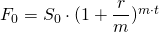In case of continiously compounded interest, from now on used a standard, the formula becomes: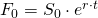## Value forward contract

The value of the contract at a certain time can be calculated in 2 ways. The first way determines the new forward price and discounting the difference with the initial forward price till today. The second possibility is by substracting the present value of the initial forward price from the spot price observed now.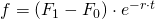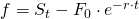## Forward price and dividends

In case of dividends, the forward price needs to be adjusted for future income streams. This can be done by either substracting from the spot price the present value of the dividends as shown below. The dividends in this case a discrete, meaning that you now when and how much you’re receiving.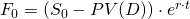Another possiblity is by calculating a dividend yield. This method implies that you are continiously earning money from you investments. This is for example often used to calculate the forward price of an index, which includes many shares.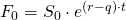## Value forward contract and dividends

In case of dividends, the value of the forward contract does not change when using the first valuaing method.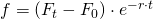In case of using the second method, it is needed to adjust the current spot price for any income earned, either discreet or continiously.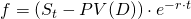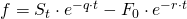## Summary

A forward contract is an obligation to buy or sell a certain asset in the future for a price fixed today. Determining the spot price, risk free rate, dividends and investment period are key to calculate the forward price and value of the forward contract.

### Forward contract Excel implementation

Need to have more insights? Download our free excel file: forward contract.